Last update: May 18, 2012

## 3. System Identification/Plant Model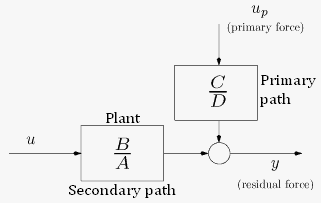Figure 3.1 System's bloc diagram of the system.
The block diagram of the active suspension system is presented in the above Figure 3.1. Two models for the system can be identified, corresponding to the primary and secondary path. Here up denotes the input of the primary path (excitation of the shaker), u is the input of the secondary path (proportional to the inertial actuator input) and y is the system output (residual force). The sampling frequency for data acquisition is Fs = 800 Hz. The best models have been obtained as follows:
• Primary path: open loop identification using ERLS
• Secondary path: identification in closed loop using a RS controller with the excitation added to the plant input (for more details see I.D. Landau, G. Zito, Digital Control Systems)
The models are available in the following files:
1. model_prim.mat
2. model_sec.mat
3. RS_contr_sec.mat (the controller used for the closed loop identification)
In Fig. 3.2, real time tests are shown in open and in closed loop for a controller designed to reject one sinusoidal disturbance. Fig. 3.3 shows the simulation results for the same configuration. A very good coherence can be observed between simulations and real time results.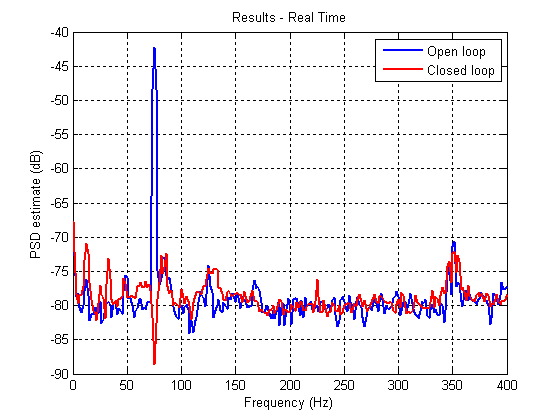Figure 3.2 Real Time results.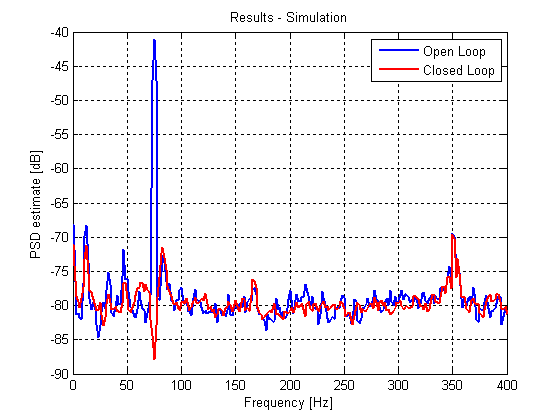Figure 3.3 Simulation results.
A non parametric model of the primary path can be identified by the spectral analysis method. This analysis shows that the model contains several high-resonant modes. The magnitude of the model frequency characteristic for the primary and the secondary paths are shown next in the Figures 3.4 and 3.5, respectively. The frequency characteristics of the identified parametric models are also shown.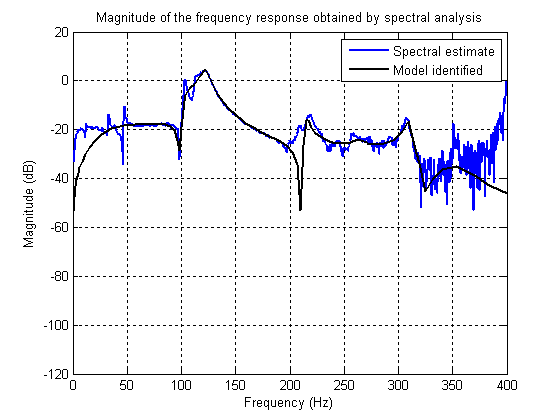Figure 3.4 Primary Path.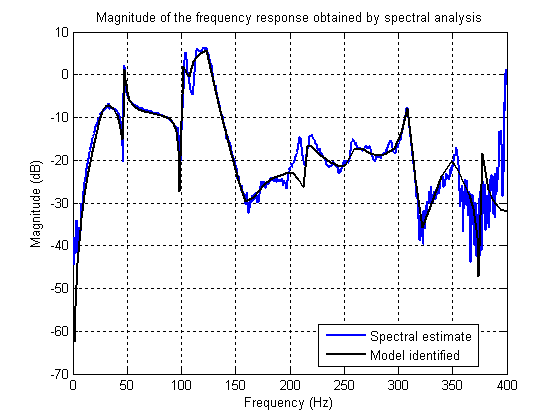Figure 3.5 Secondary Path.
Data used for system identification are given in section Data acquisition.

Continuous-time model: approximate continuous-time models of the plant can be obtained by conversion of discrete-time identified models using the zero-order hold method or from the estimated frequency characteristics.

Warning: The participants should be prepared to provide an experimental protocol for plant model identification in order to tune their control if necessary.